## 2.1Pressure

The equations of ﬂuid dynamics in CFD treat the ﬂuid as a continuous medium, or continuum. It is continuous in the sense that we consider the ﬂuid as having no “empty space” by ignoring its molecular nature. We assume it has properties that vary from point to point and are continuous throughout the solution domain, and whose derivatives are also continuous.

Pressure is an important property of a ﬂuid, denoted by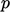. It describes the amount of force per unit surface area which acts on a surface, in the direction perpendicular to the surface.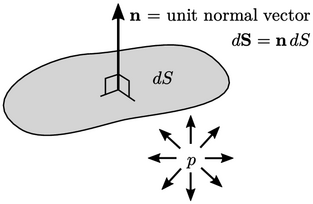Pressureis a scalar that produces a force vector with direction normal to the surface. When pressure is applied to one side of a segment of surface with area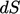, the force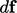points away from that side by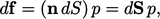(2.3)
where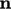is the vector of unit length, normal to the surface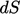. The term vector denotes a geometric entity with magnitude and direction; a surface can be represented by a surface area vector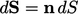of magnitudeand direction.

While pressure exerts a force on a surface, the ﬂuid experiences a force which is compressive in nature, assuming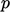is positive.

Pressure is measured in SI units of pascal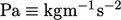. From Sec. 2.13 onwards, however, we generally useto represent the kinematic pressure, in units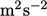, obtained by dividing the true pressure by a constant density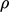.

### Scalar ﬁelds

The majority of properties, e.g. pressure, temperature, energy, density, volume, etc., can be represented by a single number, or scalar. A scalar ﬁeld describes a scalar property, e.g. pressure, which varies from point to point across some spatial domain.

Point locations can be deﬁned in any co-ordinate system of axes, e.g. Cartesian (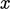,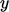,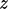), and in any orientation. A scalar ﬁeld is invariant, meaning the scalar values are the same irrespective of the co-ordinate system used.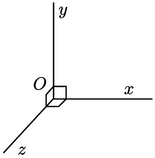In this book, space and ﬁelds will be described in a co-ordinate system with right-handed rectangular Cartesian axes. The axes are constructed by deﬁning an originfrom which three lines are drawn at right angles to each other, termed the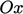,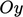,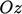axes. A right-handed set of axes requires that looking down the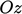axis with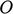nearest, an arc from the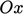axis to the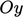axis is in a clockwise sense.

Notes on CFD: General Principles - 2.1 Pressure